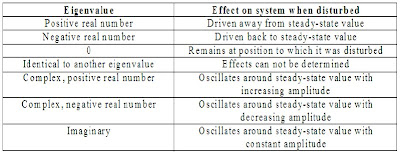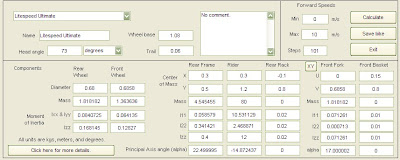## Friday, September 18, 2009

### Dynamic Stability Of Bicycle Design : Part 3

Continued from Part 2.

Hello bike nerds! Before you engage yourself in another installment in my series on bicycle stability, have a look at this clip from The Daily Planet shown on Discovery Channel Canada. The individual interviewed is Arend Schwab, a mechanical engineer with Delft University of Technology.

Modeling For Stability Analysis

The bicycle is a complex system to analyze. In Part 2, we talked a little about modeling the idealized passive rider-bicycle system, simplifying many things through assumptions but still capturing enough detail to go ahead with a reasonable analysis. The full analysis, particularly that involving the derivation of the equations of motion, is beyond the scope of this blog.

But to put it in simple words, what the analysis yields are two coupled second order, non-linear, differential equations in lean and steer. Then, these equations are linearized through a carefully followed algorithm to give us an eigenvalue problem. The eigenvalues gained from the characteristic equation help us assess the stability of the modes of bicycle motion. Eigenvalues are the cool numbers that give us an idea of the stability of the engineered system when the system is disturbed.Fig 3 : What eigenvalues tell us about stability. To make an engineered system stable, we're all interested in attaining negative real numbers for eigenvalues. Stable motion of a bicycle has negative, real, eigenvalues. Courtesy : University of Michigan, Dynamics And Controls

Many years of research has allowed us to understand the nature of the bicycle's linearized equation of motion (LEOM). The LEOM, expressed in terms of small changes in the lateral degrees of freedom being the rear frame, roll angle ф and the steering angle δ, from upright straight ahead configuration at a forward speed v, looks like this in matrix form :

where

M = symmetric mass matrix which gives the kinetic energy of bicycle system at 0 forward speed
C1 = damping matrix, proportional to forward speed v
K0 = first component of stiffness matrix, which when combined with 'g' yields a symmetric quantity proportional to gravitational acceleration and can be used to calculate changes in potential energy
K2 = second component of stiffness matrix, which when combined with the square of forward speed, v, gives a quadratic quantity in forward speed and is due to centrifugal effects.
f = applied forces

Consider each of these matrices as packages and the contents of these packages would be specific combinations of the bicycle's design parameters shown in Fig 2 of Part 2. To know what goes where in these matrices, you need to read this paper from Delft.

Finally, the time varying variables in the LEOM are :

ф = roll angle
δ = steering angle
Tф = action-reaction roll moment between fixed space and rear frame due to external causes like wind or lateral pushing force from behind.
Tδ = action-reaction steering moment, torqued by rider's hands.

Because we're analyzing a passive, uncontrolled bicycle, both these moments are taken to be 0. The characteristic equation is then the determinant of the equation in Fig 4 which gives us the eigenvalues of the problem. Eigenvalues are the exponential part of the solution to the differential equations of motion and as said before, help in stability analysis.

Computer Program To Plot Eigenvalues

Solving all this by hand takes pages of tedious work. If we can program these rules into a computer, it can quickly solve the characteristic equation. The input for the program would be all the bicycle's design parameters. The output would be the eigenvalues. We can even tell the computer to plot them for us as a function of forward speed v, for any particular bicycle configuration that we provide.

That's exactly what JBike6 does. It is a program written in MATLAB, a collaborated work between Delft University of Technology and Cornell. For small values of steer and lean, the program is perfectly accurate. Jim Papadopoulos, a contributor to JBike6, is also the co-author of the book, Bicycling Science (I had interviewed the main author, Prof. David Gordon Wilson from MIT earlier this year).

As the main illustration for today, I pull up an example bike, already provided in the program. It is a Litespeed Ultimate bicycle and Fig 7 shows its design parameters, all values in metric units.Fig 7 : Litespeed Ultimate's 25+ design parameters entered as inputs to program. Click to zoom in.

After checking these boxes, I hit the calculate button on the upper right hand side. The program solves the linearized eigenvalue problem and gives me 4 generalized eigenvalues. It plots these values on the y-axis as a function of forward speed, v on the x-axis.

The above plot tells me something about the stability of this bicycle as a function of speed. To understand what's going on, let us remember the information about eigenvalues in Fig 3 and commit it to mind, or go back and refer to it. Now read the plot in Fig 8 slowly from left to right in the order of increasing speed v. We'll take it piece by piece.

Speed Range Of Interest : 0-0.5 m/s (0-1.118 mph)
Motion: Capsize
Nature : Stable, non-oscillatory capsize

What would you expect from a bike standing still or nearly so? It will simply flop over. How do we know this? The fact that the plot yielded large positive eigenvalues or real numbers tells us right away that this is a very unstable motion. Two positive and negative pairs of roots correspond to both falling and uprighting of the bicycle. One pair corresponds to when the steering is turning toward the lean; the other when it is turning opposite to lean. Since there are no imaginary parts of eigenvalues, it tells us that this capsize motion is non-oscillating, like I mentioned in Part 2.

Speed Of Interest : 0.5-1 m/s (1.118-2.237 mph)
Motion: Transition to weave
Nature : Unstable, oscillatory
weave with stable capsize

As the forward speed v is increased from 0.5m/s to slightly more, two real eigenvalues (in blue) become identical, coalesce and form a conjugated pair, which is where oscillatory weave motion actually shows its face. In this oscillation, the bicycle sways about the headed direction.

Speed Range Of Interest : 1-4.8539 m/s (2.237-10.858 mph)
Motion: Weave+Capsize
Nature : Transition to stable weave, with stable capsize and oscillation

The positive eigenvalues tend to decrease in magnitude, so the motion is tending towards stability. Eigenvalues with imaginary parts lead to oscillation with increasing frequency, and the rate of increase is rapid at first, but then slows. The bike will weave back and forth one or more times before falling over.

Speed Range Of Interest : 4.8539 m/s (10.858 mph)
Motion: Weave + Capsize
Nature : Weave speed critical point, with stable capsize

Weave speed is that speed at which weave does not grow or decay, as can be seen by the eigenvalue crossing 0. Hence, weave speed is 4.8539 m/s. It is stable and forms the lower stability range bound for this bicycle. Eigenvalues corresponding to imaginary parts is oscillating motion. Beyond this point, weave is stable until infinity.

Speed Range Of Interest : 4.8539-7.0994 m/s (10.858-15.880 mph)
Motion: Weave + Capsize
Nature : Asymptotically stable behavior

This speed range is the stable range for the bicycle, as the eigenvalues corresponding to both weave and capsize have no positive numbers. The bike will weave back and forth, less so each time, and eventually roll straight ahead, although not necessarily in the original direction.

Speed Range Of Interest : 7.0994 m/s (15.880 mph)
Motion: Weave + Capsize
Nature : Capsize speed critical point, with stable weave

Capsize speed is that speed at which capsize does not grow or decay, as can be seen by the eigenvalue which is at 0. Hence, capsize speed is 4.8539 m/s. It forms the upper stability range bound for this bicycle. Crossing this point gets us over the stable range.

Speed Range Of Interest : Greater than 7.0994m/s (v>15.880 mph)
Motion: Weave + Capsize
Nature : Stable weave with unstable capsize

Small positive eigenvalue for capsize gets it into unstable mode. Eigenvalues with imaginary parts, but whose real component is much smaller than the positive eigenvalue overwhelms oscillations. The bike slowly leans farther and farther to one side, without oscillation, until it finally falls over.

Thus, stable speed range for an uncontrolled Litespeed Ultimate is between 10.858-15.880 mph, but for all practical purposes, we could say it becomes easily balanced above 2 m/s. One important thing to realize is that capsize instability in these regions is very slow and thus can be easily corrected by a controlling rider. Also note that all this time, we have deliberately avoided talking about wobble. Wobble is complex and cannot be described without analyzing tire dynamics. This is an on-going study in bicycle dynamics.

In the final series to come shortly, I'll show you a cool peice of literature one of my readers has authored from which we might be able to study how changing the parameters in bicycle design affects the modes of bicycle motion. Now you can all take a deep sigh and have a good weekend.